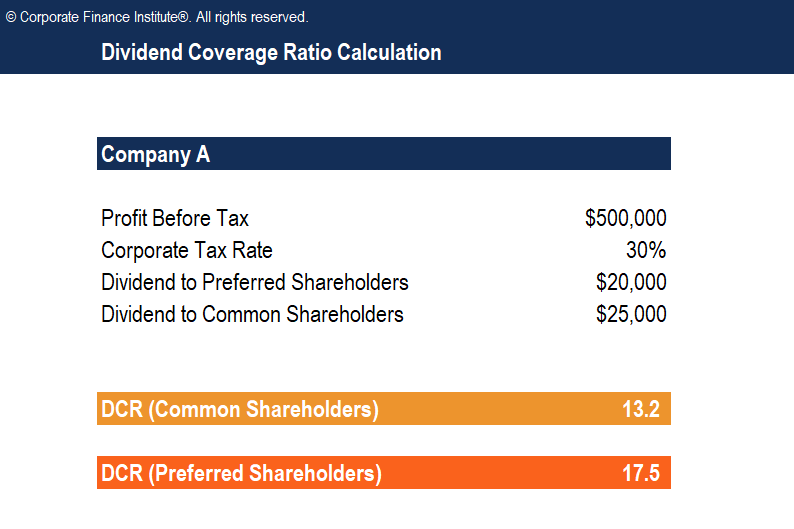# Dividend Coverage Ratio Template

## Dividend Coverage Ratio Template

This Dividend Coverage Ratio Template will show you the calculation for dividend coverage ratios for common shareholders and preferred shareholders.

Below is the screenshot of the template:### What is Dividend Coverage Ratio (DCR)?

The Dividend Coverage Ratio, also known as dividend cover, is a financial metric that measures the number of times that a company can pay dividends to its shareholders. The dividend coverage ratio is the ratio of the company’s net income divided by the dividend paid to shareholders.

### Dividend Coverage Ratio Formula

The general formula for calculating DCR is as follows:

Dividend Coverage Ratio = Net income / Dividend declared

Where:

• Net income is the earnings after all expenses, including taxes, are paid
• Dividend declared is the amount of dividend entitled to shareholders

There are also some modified versions of the dividend coverage ratio, which will be discussed below.

The first variation is used to determine the number of times a company can pay dividends to common shareholders when the company also has preferred shares to take into consideration.

The formula is:

DCR = (Net income – Required preferred dividend payments) / Dividends declared to common shareholders

This variation can also be used to determine the number of times a company can pay dividends to preferred shareholders:

The formula is:

DCR = Net income / Dividends declared to preferred shareholders

More Free Templates

For more resources, check out our business templates library to download numerous free Excel modeling, PowerPoint presentation and Word document templates.

• Excel Modeling Templates
• PowerPoint Presentation Templates
• Transaction Document Templates

### Financial Analyst Certification

Become a certified Financial Modeling and Valuation Analyst (FMVA)® by completing CFI’s online financial modeling classes and training program!